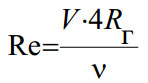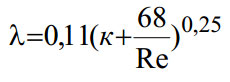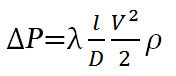# Pressure loss calculator, head loss in pipe

## Characteristics of the pipeline

Diameter: mm

The length of pipe: m

Flow: l/min

Fluid (temperature 20° C):
Water
Benzine
Petroleum
Kerosene
Hydraulic oil

Piping Material:
Steel
Cast iron
Copper
Aluminum
Rubber
Concrete
Polypropylene

## Pressure drop calculation algorithm

Checking calculation results.

Calculate values:
• Mean velocity of the fluid
•Q - volumetric flow rate, m3/s, A - pipe's cross-sectional area, m2, A=πd2/4, d - pipe bore, m
• Reynolds number Re
•V - mean velocity of the fluid, m/s, d - diameter, m, ν - kinematic viscosity, m2/c, Rr - hydraulic radius, for round pipe R=d/4, d - diameter, m

Darcy friction factor formula may be applicable depends upon the type of flow that exists.

• The Darcy friction factor for laminar flow Re<2000 is given by the following formula:
•• Which friction factor for transition between laminar and turbulent flow 2000<Re<4000 - is given by the following formula:
•• The Darcy friction factor for turbulent flow Re>4000 is given by the following formula.
• к=Δ/d, Δ - absolute roughness coefficient.The head loss in a pipe, tube or duct can be calculated with the Darcy-Weisbach equation### The pressure drop calculation

The pressure drop can be calculated with the Darcy-Weisbach equation.ρ - density of the liquid kg/m3, g - acceleration of gravity

Languages
Spanish (ES) Deutsch (DE)
Featured articles